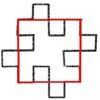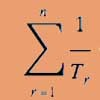# Resources tagged with: Limits

Filter by: Content type:
Age range:
Challenge level:

There are 20 NRICH Mathematical resources connected to Limits, you may find related items under Calculus.

Broad Topics > Calculus > Limits### Spokes

##### Age 16 to 18Challenge Level

Draw three equal line segments in a unit circle to divide the circle into four parts of equal area.### Exponential Trend

##### Age 16 to 18Challenge Level

Find all the turning points of y=x^{1/x} for x>0 and decide whether each is a maximum or minimum. Give a sketch of the graph.### Discrete Trends

##### Age 16 to 18Challenge Level

Find the maximum value of n to the power 1/n and prove that it is a maximum.### Witch of Agnesi

##### Age 16 to 18Challenge Level

Sketch the members of the family of graphs given by y = a^3/(x^2+a^2) for a=1, 2 and 3.### There's a Limit

##### Age 14 to 18Challenge Level

Explore the continued fraction: 2+3/(2+3/(2+3/2+...)) What do you notice when successive terms are taken? What happens to the terms if the fraction goes on indefinitely?### Rain or Shine

##### Age 16 to 18Challenge Level

Predict future weather using the probability that tomorrow is wet given today is wet and the probability that tomorrow is wet given that today is dry.### Converging Product

##### Age 16 to 18Challenge Level

In the limit you get the sum of an infinite geometric series. What about an infinite product (1+x)(1+x^2)(1+x^4)... ?### Over the Pole

##### Age 16 to 18Challenge Level

Two places are diametrically opposite each other on the same line of latitude. Compare the distances between them travelling along the line of latitude and travelling over the nearest pole.### Production Equation

##### Age 16 to 18Challenge Level

Each week a company produces X units and sells p per cent of its stock. How should the company plan its warehouse space?### Resistance

##### Age 16 to 18Challenge Level

Find the equation from which to calculate the resistance of an infinite network of resistances.### Squareflake

##### Age 16 to 18Challenge Level

A finite area inside and infinite skin! You can paint the interior of this fractal with a small tin of paint but you could never get enough paint to paint the edge.### Golden Fractions

##### Age 16 to 18Challenge Level

Find the link between a sequence of continued fractions and the ratio of succesive Fibonacci numbers.### Golden Eggs

##### Age 16 to 18Challenge Level

Find a connection between the shape of a special ellipse and an infinite string of nested square roots.### Fractional Calculus III

##### Age 16 to 18

Fractional calculus is a generalisation of ordinary calculus where you can differentiate n times when n is not a whole number.### Fractional Calculus II

##### Age 16 to 18

Here explore some ideas of how the definitions and methods of calculus change if you integrate or differentiate n times when n is not a whole number.### Fractional Calculus I

##### Age 16 to 18

You can differentiate and integrate n times but what if n is not a whole number? This generalisation of calculus was introduced and discussed on askNRICH by some school students.### Squaring the Circle and Circling the Square

##### Age 14 to 16Challenge Level

If you continue the pattern, can you predict what each of the following areas will be? Try to explain your prediction.### Lower Bound

##### Age 14 to 16Challenge Level

What would you get if you continued this sequence of fraction sums? 1/2 + 2/1 = 2/3 + 3/2 = 3/4 + 4/3 =### Reciprocal Triangles

##### Age 16 to 18Challenge Level

Prove that the sum of the reciprocals of the first n triangular numbers gets closer and closer to 2 as n grows.### Triangle Incircle Iteration

##### Age 14 to 16Challenge Level

Keep constructing triangles in the incircle of the previous triangle. What happens?